# bLSAG ring signatures overview

2022-07-20

Note: I’m not a mathematician, I’m just an amateur on math. These notes are just an attempt to try to sort the notes that I took while learning abut bLSAG.

bLSAG: Back’s Linkable Spontaneous Anonymous Group signatures

• signer ambiguity
• unforgeability

### Setup

Let $$G$$ be the generator of an EC group. We use a hash function $$\mathcal{H}_p$$, which maps to curve points in EC, and a normal hash $$\mathcal{H}_n$$, which maps to $$\mathbb{Z}_p$$. Signer’s key pair: $$k_{\pi}$$, s.t. $$K_{\pi} = k_{\pi} \cdot G \in \mathcal{R}$$, with secret index $$\pi$$. Set of Public Keys: $$\mathcal{R} = \{ K_1, K_2, \ldots, K_n \}$$

def new_key():
k = F.random_element()
K = g * k # g is the generator of the EC group
return K


### Signature

1. compute key image: $$\tilde{K} = k_{\pi} \mathcal{H_p} ( K_{\pi}) \in G$$

key_image = k * hashToPoint(K)

2. Generate $$\alpha \in^R \mathbb{Z}_p$$, and $$r_i \in^R \mathbb{Z}_p$$, for $$i \in \{1, 2, \ldots, n \}$$, with $$i \neq \pi$$

• $$r_i$$ is used for the fake responses

a = F.random_element()
r = [None] * len(R)
for i in range(0, len(R)):
if i==pi:
continue

r[i] = mod(F.random_element(), p)

3. Compute $$c_{\pi + 1} = \mathcal{H}_n ( m, [\alpha G], [\alpha \mathcal{H}_p(K_{\pi})])$$

c[pi1] = hash(R, m, a * g, hashToPoint(R[pi]) * a, p)

4. for $$i=\pi + 1, \pi +2, \ldots, n, 1, 2, \ldots, \pi -1$$, calculate, replacing $$n+1 \rightarrow 1$$ $$$c_{i+1} = \mathcal{H}_n (m, [r_i G + c_i K_i], [r_i \mathcal{H}_p (K_i) + c_i \tilde{K}])$$$

• Notice that (from step 3 & 4):
$$\alpha \mathcal{H}_p (K_{\pi}) = r_{\pi} \mathcal{H}_p (K_{\pi}) + c_{\pi} \cdot (\tilde{K})$$,
where $$\tilde{K}= k_{\pi} \mathcal{H_p} ( K_{\pi})$$, so:
$$\alpha \mathcal{H}_p (K_{\pi}) = r_{\pi} \mathcal{H}_p (K_{\pi}) + c_{\pi} \cdot (k_{\pi} \mathcal{H}_p(K_{\pi}))$$
which is equal to,
$$\alpha \cdot \mathcal{H}_p (K_{\pi}) = (r_{\pi} + c_{\pi} \cdot k_{\pi}) \cdot \mathcal{H}_p(K_{\pi})$$
From where we can see: $$\alpha = r_{\pi} + c_{\pi} \cdot k_{\pi}$$
which we can rearrange to $$r_{\pi} = \alpha - c_{\pi} \cdot k_{\pi}$$.

for j in range(0, len(R)-1):
i = mod(pi1+j, len(R))
i1 = mod(pi1+j +1, len(R))

c[i1] = hash(R, m, r[i] * g + c[i] * R[i],
r[i] * hashToPoint(R[i]) + c[i] * key_image, p)

5. Define $$r_{\pi} = \alpha - c_{\pi} k_{\pi} \mod{p}$$

r[pi] = mod(a - c[pi] * k, p)


Signature: $$\sigma(m) = (c_1, r_1, \ldots, r_n)$$, with key image $$\tilde{K}$$ and ring $$\mathcal{R}$$. - $$len(\sigma(m)) = 1+n$$

return [c, r]


#### Step by step (simplified):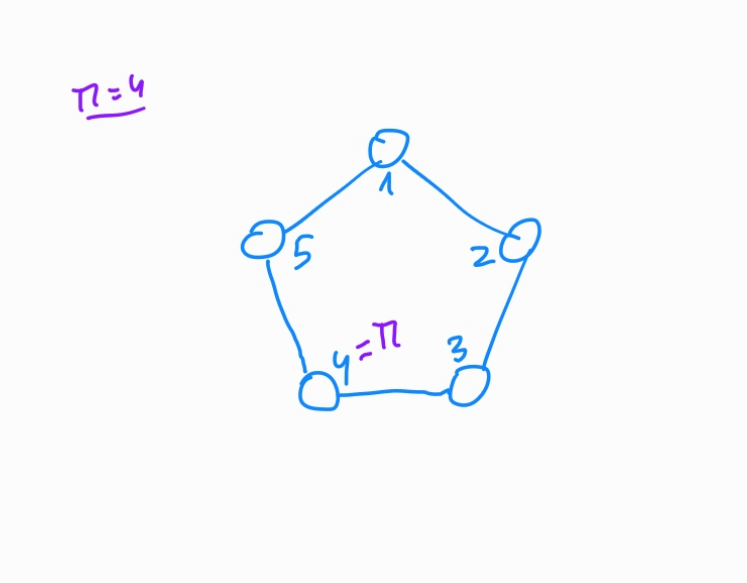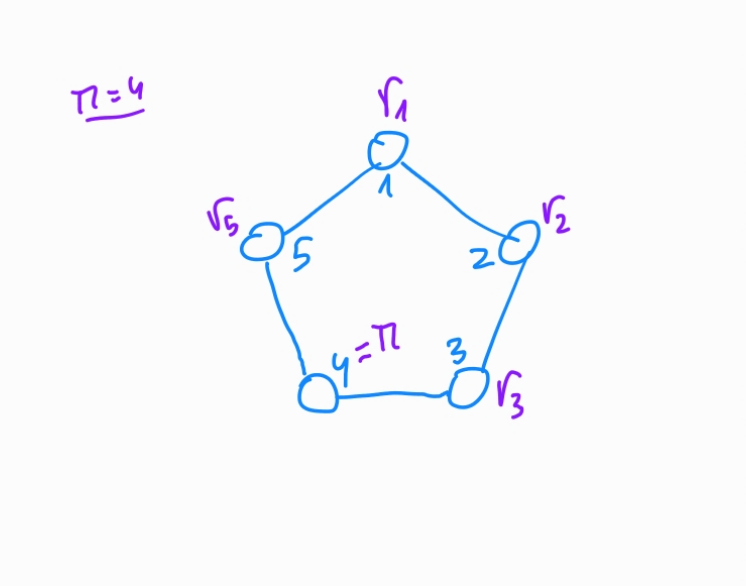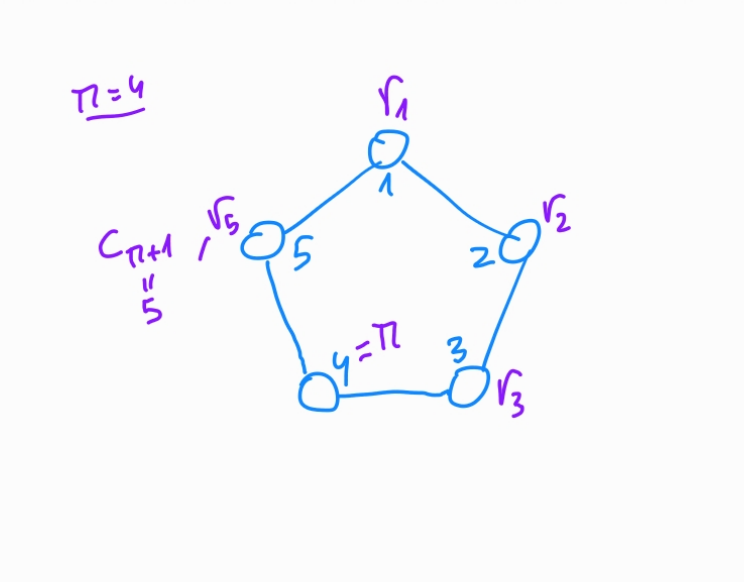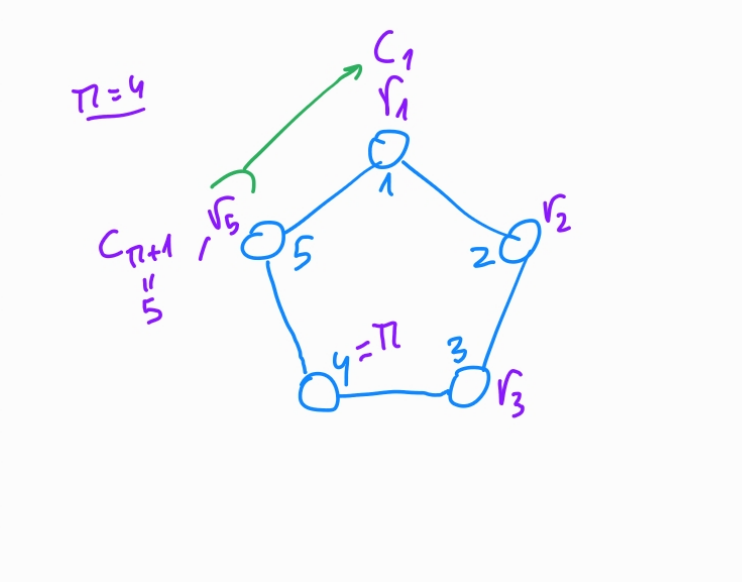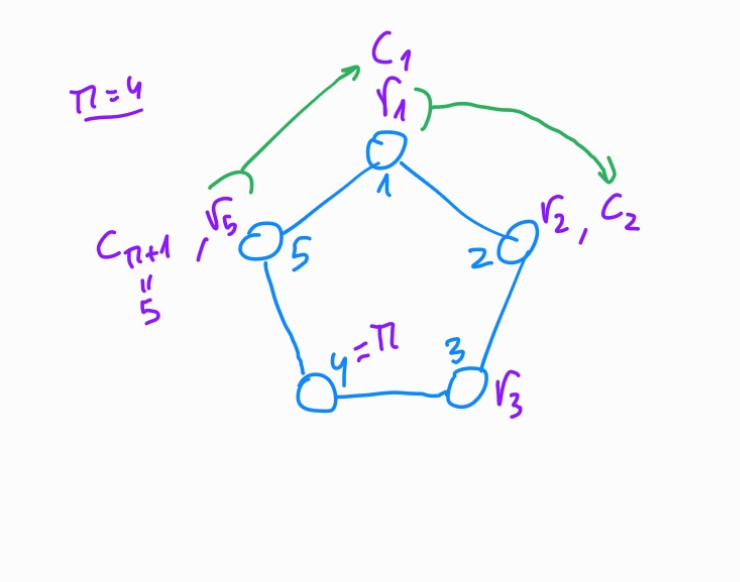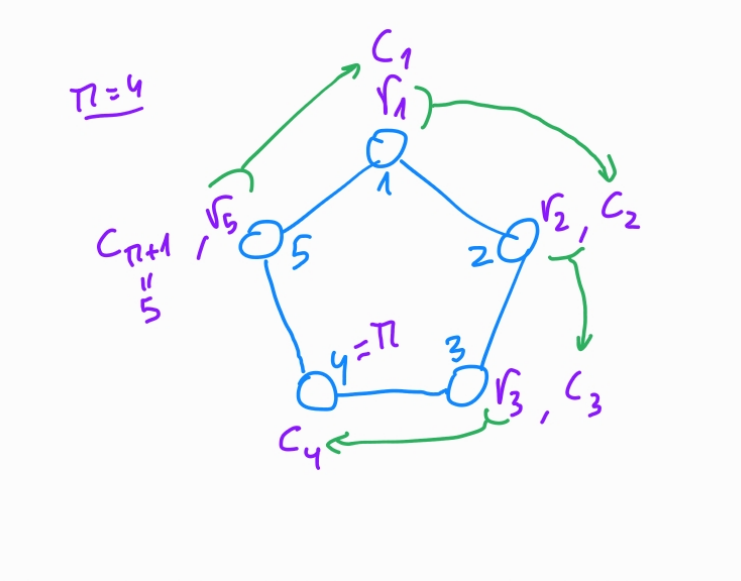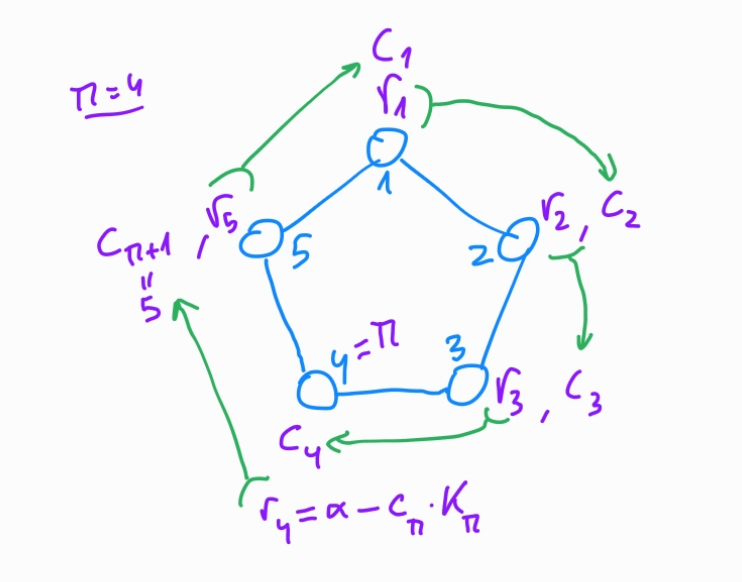• Generate $r_i \in^R \mathbb{Z_p}$
• Compute $c_{i+1}$ from $r_i$
• Link $r_{\pi}$ with $c_{\pi}$

You can scroll down the images through the step-by-step diagrams.

It reminds in some way to the approach to close a box like the one in the picture: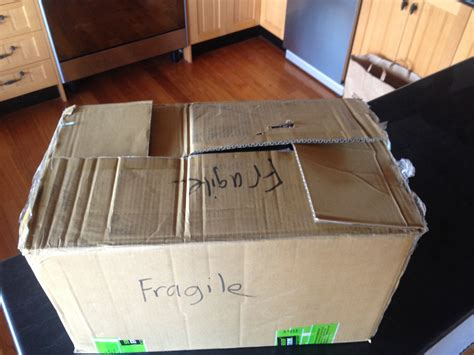### Verification

1. check $$p \tilde{K} \stackrel{?}{=} 0$$

• to ensure that $$\tilde{K} \in G$$ (and not in a cofactor group of $$G$$)
2. for $$i = 1, 2, \ldots, n$$, replacing $$n+1 \rightarrow 1$$ $$$c'_{i+1} = \mathcal{H}_n (m, [r_i G + c_i K_i], [r_i \mathcal{H}_p (K_i) + c_i \tilde{K}])$$$

3. check $$c_1 \stackrel{?}{=} c'_i$$

c = c1
for j in range(0, len(R)):
i = mod(j, len(R))
i1 = mod(j+1, len(R))
c[i1] = hash(R, m, r[i] * g + c[i] * R[i],
r[i] * hashToPoint(R[i]) + c[i] * key_image, p)

assert c1 == c